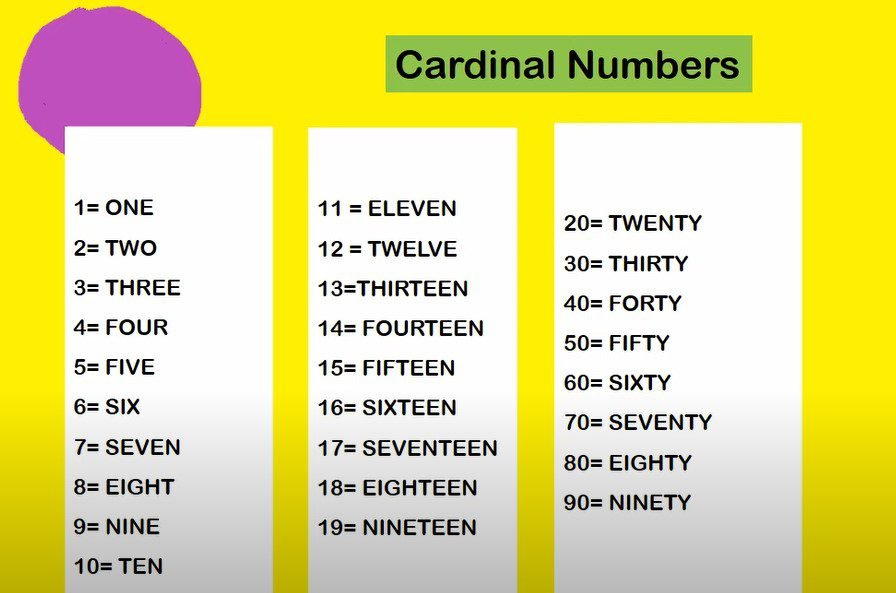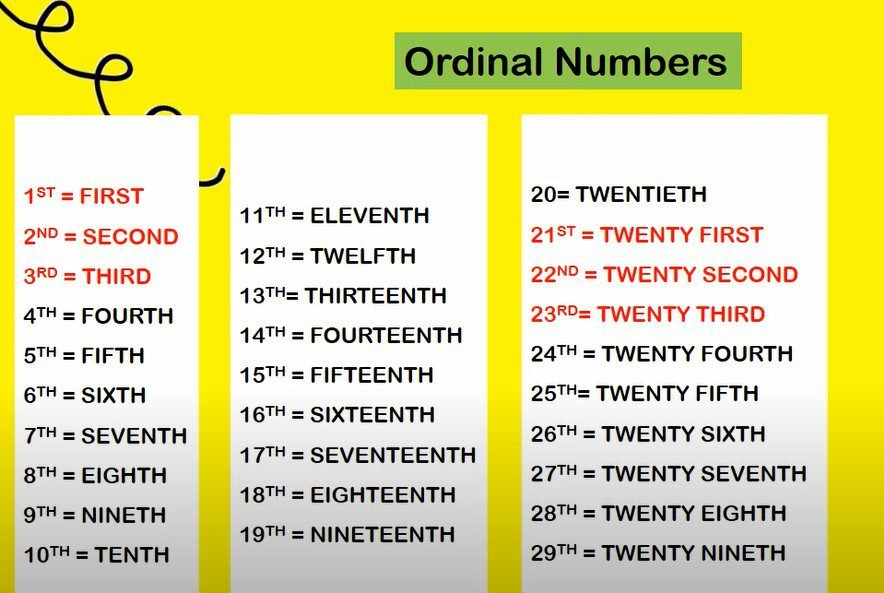# Diference of Cardinal and Ordinal Numbers

Using numbers in English is not the same as numbers in other country. In English, the terms cardinal and ordinal numbers are known. What are cardinal and ordinal numbers? Check out this study.

In English lessons, there are two types of numbers. The first number is known as Cardinal Numbers, while the second number is known as Ordinal Numbers. What’s the difference between the two?

## Understanding Cardinal and Ordinal NumbersCardinal numbers are numbers used to describe numbers in integers. The ordinal numbers are numbers used to describe positions. To make it easier to understand, consider the following picture.

The picture above, on the left is an example of a Cardinal number, which is also a number that is used to express the rounded quantity of the object in question. The right part is an example of an Ordinal number which is commonly used to express a sequence or a stage.

If you’ve ever watched MotoGP, at the end of the race, the rankings achieved by each racer will be announced. That’s where Ordinal numbers play a role because the numbers used are intended to tell the position and not the number.

An example of an Ordinal Number is as below:

• 1st Marquez
• 2nd Dovizioso
• 3rd Vinales

Meanwhile, if you are in a market and asked by the seller how much watermelon you want to buy, you need to answer it using the Cardinal number.

An example of a Cardinal Number is as follows:

“Hey, bro. How many watermelons you want to buy?”

“5 watermelons”

It’s impossible for you to want to answer using the sentence “5th watermelon”?

## Use of Cardinal and Ordinal Numbers

Earlier, it was discussed that the cardinal is used to express the number and the ordinal to announce the position. Both cardinal and ordinal have their respective uses that you need to know so that there are no mistakes in their use.

## Cardinal NumbersCardinal numbers are used to indicate the number and are usually used to answer the question “how many?”. One thing to keep in mind is that cardinal numbers are always whole: 1, 2, 3, etc. and not fractions or numbers other than whole numbers. It’s simple, isn’t it? Haha. Yes indeed. A bit tricky is for ordinal numbers.

## Ordinal Numbers• Level

For example, if you want to tell uncle that you are now in grade 2 of high school, then you need to say it using ordinal numbers. Ordinal numbers are also used to refer to the levels in a building.

Example :

2nd year of senior high school

37th floor

• Date

Ordinal numbers are also used to express dates. For example August 17th, June 3rd, etc.

• Order

If you like making video tutorials on cooking, makeup, and so on, you need to use ordinal numbers to sort each step you want to share. For example this:

First, turn on the stove

Second, put your pot on the stove

Third, turn off the stove

• The denominator of the fraction

The denominator is the number at the bottom of the fraction and the numerator is the number at the top. Well, in English, the denominator uses ordinal numbers. For example, to express then we use the three fourth sentence.

So in conclusion, in ordinal numbers, there are four endings used, namely: -st, -nd, -rd, -th. The four endings must be remembered, Friend. The four suffixes are used to indicate the unit number. But you need to pay attention, Friend . If you find the number 33, add the suffix -rd instead of -th. So you guys read it 33rd, thirthy third not thirthy thirth. Similarly, 41st is read as forty first not forty forth, and so on.

### Related Post